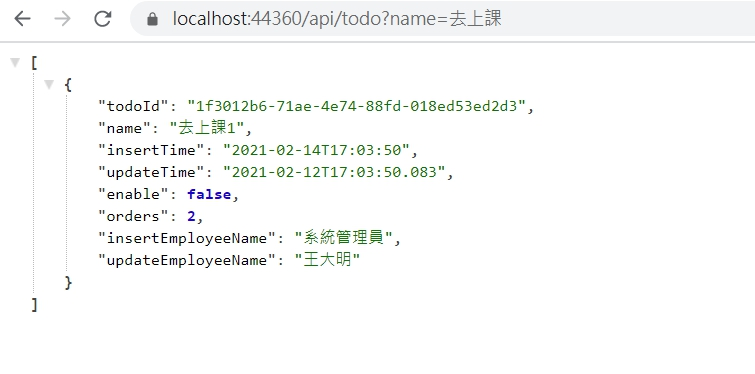# 4-2.ASP.NET Core Web API 入門教學 - 關鍵字搜尋

ASP.NET Core Web API 入門教學

``````[HttpGet]
public IEnumerable<TodoListSelectDto> Get(string name, bool? enable, DateTime? InsertTime)
{
var result = _todoContext.TodoLists
.Select(a => new TodoListSelectDto
{
Enable = a.Enable,
InsertEmployeeName = a.InsertEmployee.Name,
InsertTime = a.InsertTime,
Name = a.Name,
Orders = a.Orders,
TodoId = a.TodoId,
UpdateEmployeeName = a.UpdateEmployee.Name,
UpdateTime = a.UpdateTime
});
return result;
}``````

``````if (!string.IsNullOrWhiteSpace(name))
{
result = result.Where(a => a.Name.Contains(name));
}``````

``````if (enable != null)
{
result = result.Where(a => a.Enable == enable);
}``````

``````if (InsertTime != null)
{
result = result.Where(a => a.InsertTime.Date == InsertTime);
}````````````[HttpGet]
public IEnumerable<TodoListSelectDto> Get(string name, bool? enable, DateTime? InsertTime)
{
var result = _todoContext.TodoLists
.Select(a => new TodoListSelectDto
{
Enable = a.Enable,
InsertEmployeeName = a.InsertEmployee.Name,
InsertTime = a.InsertTime,
Name = a.Name,
Orders = a.Orders,
TodoId = a.TodoId,
UpdateEmployeeName = a.UpdateEmployee.Name,
UpdateTime = a.UpdateTime
});

if (!string.IsNullOrWhiteSpace(name))
{
result = result.Where(a => a.Name.Contains(name));
}

if (enable != null)
{
result = result.Where(a => a.Enable == enable);
}

if (InsertTime != null)
{
result = result.Where(a => a.InsertTime.Date == InsertTime);
}

return result;
}``````